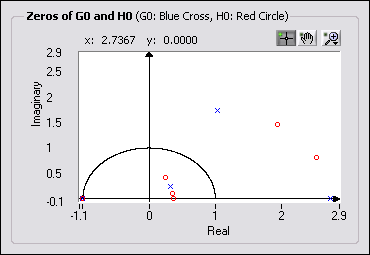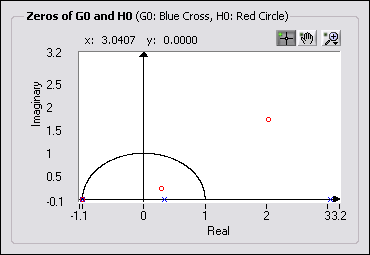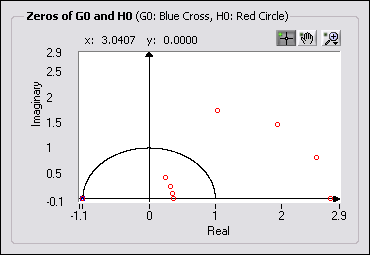# Selecting the Factorization Type for P0(z) (Advanced Signal Processing Toolkit)

LabVIEW 2014 Advanced Signal Processing Toolkit Help

Edition Date: June 2014

Part Number: 372656C-01

»View Product InfoDownload Help (Windows Only)

After you determine P0(z), the next step is to specify how P0(z) is factorized into the analysis lowpass filter G0(z) and the synthesis lowpass filter H0(z), respectively. In the Wavelet Design Express VI, use the Factorization (Type of G0) control to specify the factorization type. The factorizing process is not unique. For a given P0(z), you have the following four options for creating G0(z) and H0(z).

• Arbitrary—No specific constraints are associated with this filter. The following figure shows an example of arbitrary factorization. The blue crosses represent the zeros of G0(z), and the red circles represent the zeros of H0(z). Click on the zero you want to select to switch the zero from that of G0(z) to that of H0(z) and vice versa.• Minimum Phase—All of the zeros of G0(z) are contained inside the unit circle, as shown in the following figure. All the zeros of H0(z) are the reciprocal of the zeros of G0(z). The Wavelet Design Express VI automatically generates the zeros for H0(z) and G0(z). You cannot switch the zeros between G0(z) and H0(z). The minimum phase filter possesses minimum phase-lag. When P0(z) is maximally-flat and G0(z) is minimum phase, the resulting wavelets are the Daubechies wavelets.• Linear Phase—Any zero and its reciprocal must belong to the same filter, as shown in the following figure. When you switch a zero of G0(z) to that of H0(z), the reciprocal of the zero also switches to H0(z). When you switch a zero of H0(z) to that of G0(z), the reciprocal of the zero also switches to G0(z). This option is available only if the filter is biorthogonal.In the time domain, a linear phase implies that the coefficients of the filter are symmetric or antisymmetric. Linear phase filters have a constant group delay for all frequencies. This property is required in many signal and image feature-extraction applications, such as peak detection and image edge detection.
• B-Spline—This option is available only if Wavelet Type is Biorthogonal and P0 type is Maxflat. In this case, the analysis lowpass filter G0(z) and the synthesis lowpass filter H0(z) are defined by the following equations, respectively:

G0(z) = (1 + z–1)k

H0(z) = (1 + z–1)2p–kQ)z)

where k is specified with the Zeros at(P0) control, and p is determined by the Zero pairs at(P0) control. The Wavelet Design Express VI automatically generates the zeros of G0(z) and H0(z) based on the settings for k and p. You cannot switch the zeros between G0(z) and H0(z). The following figure shows an example of B-Spline factorization.